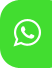## Product Detail## Ignou solved assignment for BCS-054 Computer Oriented Numerical Techniques

University  IGNOU
Service Type Solved Assignment
Course B.C.A
Semester
Short Name or Subject Code BCS-054 Computer Oriented Numerical Techniques
Product B.C.A of Solved Assignment (IGNOU)
Pattern
Price
Click to view price

This assignment has eight questions of total 80 marks. Answer all the questions. 20 marks are for viva voce. You may use illustrations and diagrams to enhance explanations. Please go through the guidelines regarding assignments given in the Programme Guide for the format of presentation. Illustrations/ examples, where-ever required, should be different from those given in the course material. You must use only simple calculator to perform the calculations.

Q1.

(a) Use the eight-decimal digit floating point representation as given in your Block 1, Unit 1, Section 1.3.1 page 29 to perform the following operations:

(i) Represent 0.0006374845 and 5749855743 as floating point numbers in normalised form using chopping for first number and rounding for second number.

(ii) Given the above two numbers what is the absolute and relative error in their representation.

(iii)Subtract the smaller number from the bigger numbers. What is the error in the resulting number?

(iv)Divide the first number by the second number. Convert the result into normalized form in the given format.

(v) Take the first number as 586309 and assume any second number to demonstrate the concepts of overflow or underflow for the given representation. (You may assume any second number to demonstrate overflow or underflow).

(vi)Explain the term bias in the context of binary floating point representation.

(b) Explain the term Unstable Algorithm and Unstable Problem with the help of one example of each other than the example given in the course material.

(c) Find the Maclaurin series for calculating (1-2x)-1 at x=0. Use first fo

ur terms of this series to calculate the value of (1-2x)-1 at any value of x. Also find the bounds of truncation error for such cases.

(d) What is Taylor's series? Explain with the help of an example. Explain the Truncation errors in this context.

Q2.

(a) Solve the system of equations

x + y + 6z = 6

7x + 3y – 4z = 4

2x – 7y + 3z = 21

using Gauss elimination method with partial pivoting. Show all the steps.

(b) Perform four iterations (rounded to four decimal places) using

(i) Jacobi Method and

(ii) Gauss-Seidel method

for the following system of equations.

4 1 -2 x 15

1 -6 2 y = -10

2 4 8 z -24

With (0) x = (0, 0, 0)T . The exact solution is (2, 1, -3)T.

Which method gives better approximation to the exact solution?

Q3

Determine the largest negative root of the following equation:

f(x) = 4x3 – 6x2 – 8

The root should be correct up to 2 decimal places, using

(a) Regula-falsi method (b) Newton-Raphson method (c) Bisection method (d) Secant method

Q4.

(a) Find Lagrange’s interpolating polynomial that fits the following data.

Hence obtain the value of f(3.5).

x 1 3 6 10

f(x) 1 7 31 91

(b) Using the Lagrange's inverse interpolation method, find the value of x when y is 7.

x 4 16 36 81

y=f(x) 1 3 5 8

Q5.

(a) The population of a State for the last 20 years is given in the following table:

Year (x) : 1998 2003 2008 2013 2018

Population(y) (in Lakhs) : 19 40 79 142 235

(i) Using Stirling's central difference formula, estimate the population for the year 2007

(ii) Using Newton’s forward formula, estimate the population for the year 2000.

(iii) Using Newton’s backward formula, estimate the population for the year 2015.

(b) Derive an expression of forward difference operator in terms of δ.

Q6.

(a) Find the values of the first and second derivatives of y = x2+x-1 for x=2.25 using the following table. Use forward difference method. Also, find Truncation Error (TE) and actual errors.

x : 2 2.5 3 3.5

y : 5.00 7.75 11.00 14.75

(b) Find the values of the first and second derivatives of y = x2+x-1 for x=2.25 from the following table using Lagrange’s interpolation formula. Compare the results with (a) part above.

x : 2 2.5 3 3.5

y : 5.00 7.75 11.00 14.75

Q7. Compute the value of the integral

∫ 0 (2x3+ 5x2 - 11) dx

By taking 12 equal subintervals using

(a) Trapezoidal Rule and then (b) Simpson's 1/3 Rule. Compare the result with the actual value.

Q8. (a) Solve the Initial Value Problem, using Euler’s Method for the differential Equation:

y = 1+x2 y, given that y(0) = 1.

Find y(1.0) taking (i) h = 0.25 and then (ii) h = 0.1

(b) Solve the following Initial Value Problem using (i)R-K method of O(h2 ) and (ii) R-K method of O(h4 )

y' = xy + x2 and y(0) = 1.

Find y(0.4) taking h = 0.2, where y' means dy/dx